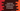# TypeScript template string examples# Introduction :

In TypeScript, we can use template strings instead of normal strings. In simple words, these are strings created using backticks or `. These are also called template literals or string literals. Template string or template literals have a lot of benefits over traditional strings that use single and double-quotes. In this post, we will learn the main advantages or main usage of template strings with examples.

## Usage of template strings :

Template strings are used mainly for three purposes :

1. Interpolation

2. Multiple lines string

3. Tagged templates

## Template string for interpolation :

String interpolation is also called string templating. If we want to create one string using different sub-strings and variables, we can use concatenation operator or +. For example :

``````let one = 1;
let two = 2;
let finalStr = "One + two = "+(one+two)+".";

console.log(finalStr);
``````

Here, we are appending strings and numbers using +. It will print :

``````One + two = 3.
``````

With template string, you can do it like below :

``````let one = 1;
let two = 2;
let finalStr = `One + two = \${one+two}.`;

console.log(finalStr);
``````

It will print the same output.

Instead of +, we have placed the string inside two backticks and placed the expression inside the interpolation \${}. Anything you placed inside the interpolation is treated as a javascript expression.

## Template string for multiline string :

If you want to create a multiline string, you can create one by adding the newline character \n like below :

``````const finalStr = "Hello\
\nWorld\
\n!!";

console.log(finalStr);
``````

And using template string :

``````const finalStr = `Hello
World
!!`;

console.log(finalStr);
``````

Clean and simple !!

## Tagged templates :

Tags are used to preprocess a string. It is a function, that processes a string and returns that string to use. We can use tags instead of creating a new function to do any changes to a string like converting the characters to uppercase or lowercase, removing special characters from a string, formatting dates or any other string operation.

The tag function consists of two arguments. The first argument is an array of substrings, i.e. the substrings in the original string. The second argument is an array of evaluated expressions. The return value of the function is used as the modified string. For example :

``````function modify(substrings, ...expressions) {
return `\${substrings}+\${substrings}+\${substrings} = \${expressions + expressions + expressions}`;
}

let first = 1;
let second = 2;
let third = 3;

let str = modify`One \${first} two \${second} three \${third}`;

console.log(str);
``````

It will print :

``````One + two + three  = 6
``````

The first argument substrings holds the strings :_One , two _ and _ three _. The second argument expressions holds the values of the expressions i.e. values of first, second and third.

### Conclusion :

Template string is more useful than the other single or double quote strings. Try to go through the examples explained above and drop one comment below if you have any queries.